# 隐写术并不是黑客特工的专属 - 我用前端实现的隐写,非常有意思 ❗

## 前言

• 文本隐写：通过某种方法竟然可以在字符串中读取和修改隐藏信息"中秋快乐"⇄"中[隐藏数据]秋快乐",点这里体验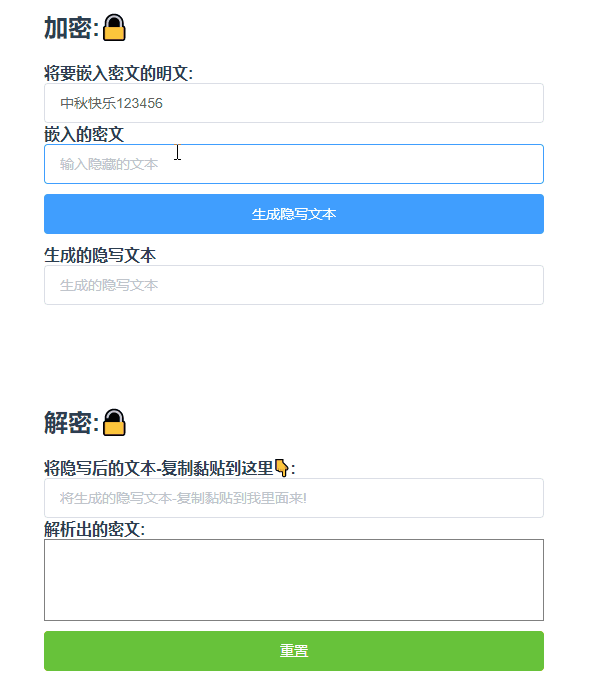• 图片隐写：在图片中添加隐藏的图文(还能往里面写文件!!!)点这里体验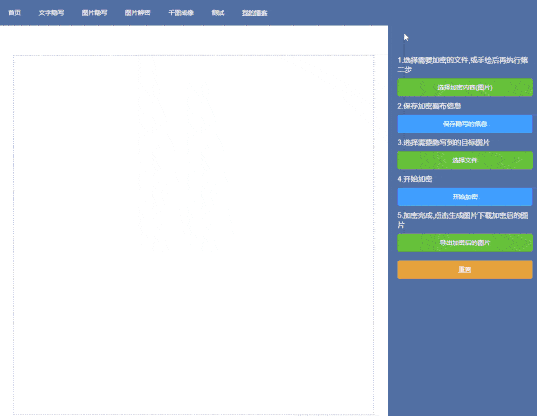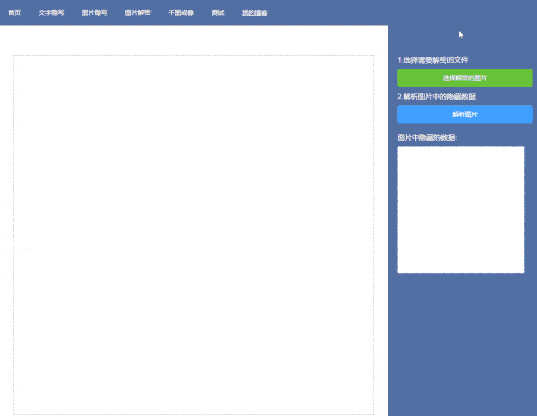## 文本隐写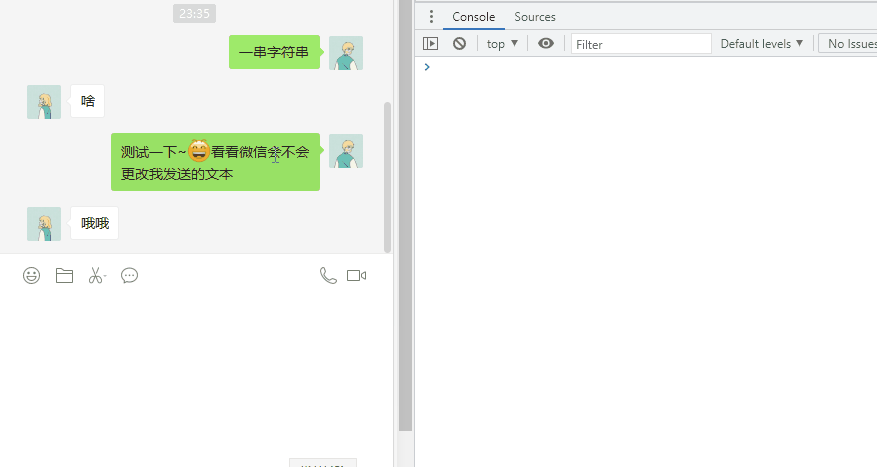### 实现原理

😀 零宽字符: 是一种不可打印的 Unicode 字符, 在浏览器等环境不可见, 但是真是存在, 获取字符串长度时也会占位置, 表示某一种控制功能的字符.

• 零宽度空格符 (zero-width space) U+200B : 用于较长单词的换行分隔
• 零宽度非断空格符 (zero width no-break space) U+FEFF : 用于阻止特定位置的换行分隔
• 零宽度连字符 (zero-width joiner) U+200D : 用于阿拉伯文与印度语系等文字中，使不会发生连字的字符间产生连字效果
• 零宽度断字符 (zero-width non-joiner) U+200C : 用于阿拉伯文，德文，印度语系等文字中，阻止会发生连字的字符间的连字效果
• 左至右符 (left-to-right mark) U+200E : 用于在混合文字方向的多种语言文本中，规定排版文字书写方向为左至右
• 右至左符 (right-to-left mark) U+200F : 用于在混合文字方向的多种语言文本中，规定排版文字书写方向为右至左

`Surrogate Pair`：是 UTF-16 中用于扩展字符而使用的编码方式，是一种采用四个字节(两个 UTF-16 编码)来表示一个字符。

Unicode 编码单元（code points）的范围从 0 到 1,114,111。开头的 128 个 Unicode 编码单元和 ASCII 字符编码一样。 如果指定的 index 小于 0 或大于字符串的长度，则 charCodeAt 返回 NaN

``````//一个字符由两个字节还是由四个字节组成的最简单方法。
"我".codePointAt(0) > 0xFFFF;//false
"A".codePointAt(0) > 0xFFFF;//false
"3".codePointAt(0) > 0xFFFF;//false

"𠮷".codePointAt(0) > 0xFFFF;//true

``````console.log(String.fromCodePoint(0x20bb7)); // "𠮷"
//或者十进制
console.log(String.fromCodePoint(134071)); // "𠮷"
//也可以多个参数
console.log(String.fromCodePoint(25105,29233,0x4f60)); // "我爱你"

`String.fromCodePoint` 方法是 ES6 新增加的特性，ES6 提供了 String.fromCodePoint()方法，可以识别大于 0xFFFF 的字符，弥补了 String.fromCharCode()方法的不足。一些老的浏览器可能还不支持。可以通过使用这段 polyfill 代码来保证浏览器的支持

### 好了,铺垫完了,开干~

``````  // 字符串转零宽字符串
function encodeStr(val = "隐藏的文字") {
return (
val
.split("")
.map((char) => char.codePointAt(0).toString(2))
.join(" ")
.split("")
.map((binaryNum) => {
if (binaryNum === "1") {
return "​"; // 零宽空格符&#8203;
} else if (binaryNum === "0") {
return "‌"; // 零宽不连字符&#8204;
} else {
return "‍"; //空格 -> 零宽连字符&#8205;
}
})
.join("‎")
);
}
console.log("str:",encodeStr(),"length:",encodeStr().length)//大家可以把这段copy到控制台执行以下看看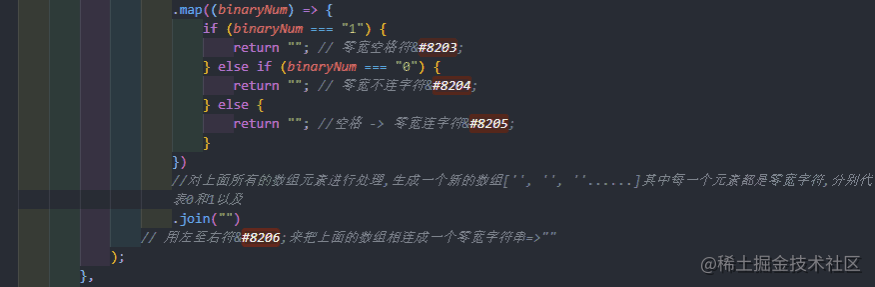``````
// 字符串转零宽字符串
encodeStr() {
this.cipherText = this.text.split("");
//在字符串中的随机一个位置插入加密文本
this.cipherText.splice(
parseInt(Math.random() * (this.text.length + 1)),
0,
//加密的文本
this.hiddenText
.split("")
//['荣', '顶' ]
.map((char) => char.codePointAt(0).toString(2))
// ['1000001101100011','1001100001110110']
.join(" ")
//"1000001101100011 1001100001110110"
.split("")
/* [ '1', '0', '0', '0',  '0', '0', '1', '1', '0', '1', '1', '0', '0',  '0', '1', '1', ' ',
'1', '0', '0', '1', '1',  '0', '0', '0', '0', '1', '1', '1', '0', '1',  '1', '0'] */
.map((binaryNum) => {
if (binaryNum === "1") {
return String.fromCharCode(8203); // 零宽空格符&#8203;
} else if (binaryNum === "0") {
return String.fromCharCode(8204); // 零宽不连字符&#8204;
} else {
return String.fromCharCode(8205); //空格 -> 零宽连字符&#8205;
}
})
//对上面所有的数组元素进行处理,生成一个新的数组['​', '​', '‌'......]其中每一个元素都是零宽字符,分别代表0和1以及
.join(String.fromCharCode(8206))
// 用左至右符&#8206;来把上面的数组相连成一个零宽字符串=>"‎​‎‌‎‌"
);
this.cipherText = this.cipherText.join("");
console.log(this.cipherText, "cipherText");
}

``````let str = "qwe12345789".split("");
//在字符串中的随机一个位置插入加密文本
str.splice(parseInt(Math.random()*(str.length+1)),0,"加密文本").join("")

``````"中秋节快乐​‎​‎‌‎​‎​‎‌‎‌‎‌‎‌‎​‎​‎​‎‌‎‌‎‌‎‍‎​‎​‎‌‎‌‎​‎‌‎​‎​‎​‎​‎‌‎‌‎‌‎‌‎‌‎‍‎​‎‌‎‌‎‌‎‌‎​‎‌‎‍‎​‎​‎​‎‌‎​‎‌‎​‎‍‎​‎​‎‌‎‌‎​‎​‎​".trim().length//114``````"点赞鼓励~😀​‎​‎​‎‌‎‌‎​‎‌‎‌‎​‎‌‎‌‎​‎‌‎‌‎‌‎‍‎​‎​‎‌‎‌‎​‎​‎​‎‌‎​‎‌‎‌‎‌‎‌‎​‎​‎‍‎​‎‌‎‌‎​‎​‎​‎​‎​‎​‎​‎‌‎‌‎‌‎‌‎​‎‍‎​‎​‎‌‎‌‎‌‎‌‎‌‎‌‎​‎​‎‌‎​‎​‎​‎​‎‍‎​‎​‎‌‎​‎​‎‌‎‌‎‌‎‌‎‌‎​‎​‎​‎​‎‌‎​‎‍‎​‎​‎‌‎​‎​‎​‎​‎‌‎‌‎‌‎‌‎‌‎‌‎‌‎‌‎‌".replace(/[^\u200b-\u200f\uFEFF\u202a-\u202e]/g, "");

``````// 零宽字符转字符串
decodeStr() {
if (!this.tempText) {
this.decodeText = "";
return;
}
let text = this.tempText.replace(/[\u200b-\u200f\uFEFF\u202a-\u202e]/g, "");
let hiddenText = this.tempText.replace(/[^\u200b-\u200f\uFEFF\u202a-\u202e]/g, "");
console.log(text, "text");
console.log(hiddenText, "hiddenText");
this.decodeText = hiddenText
.split("‎") //不是空字符串,是 &#8206;
.map((char) => {
if (char === "​" /* 不是空字符串,是&#8203; */) {
return "1";
} else if (char === "‌" /*  不是空字符串,是&#8204; */) {
return "0";
} else {
/* 是&#8205;时,用空格替换 */
return " ";
}
})
.join("")
//转数组
.split(" ")
//根据指定的 Unicode 编码中的序号值来返回一个字符串。
.map((binaryNum) => String.fromCharCode(parseInt(binaryNum, 2)))
.join("");
console.log(text + hiddenText);
},

### 演示一波### 应用场景

• 数据防爬
将零宽度字符插入文本中,干扰关键字匹配。爬虫得到的带有零宽度字符的数据会影响他们的分析，但不会影响用户的阅读数据。
• 隐藏文本信息
将自定义组合的零宽度字符插入文本中，用户复制后会携带不可见信息，达到隐藏文本信息的作用。
• 逃脱敏感词过滤
你在游戏里骂人,`你妈*`肯定是发不出去的,但是你发`你xxxxx妈yyyyy*`就不一样了 😀,这个东西还是研究起来很有意思的。
• 嵌入隐藏的代码还有很多等等等...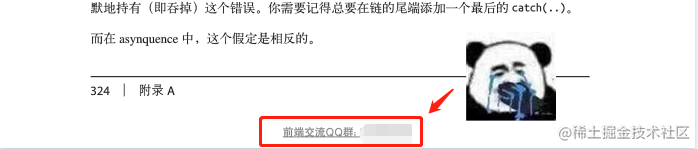### 预防零宽字符的植入

``````str.replace(/[\u200b-\u200f\uFEFF\u202a-\u202e]/g, "");

## 图片隐写

lsb 隐写很实用，算法简单，能存储的信息量也大，更何况是 CTF 比赛中的常客(起源于 1996 年 DEFCON 全球黑客大会)

### 二进制最低位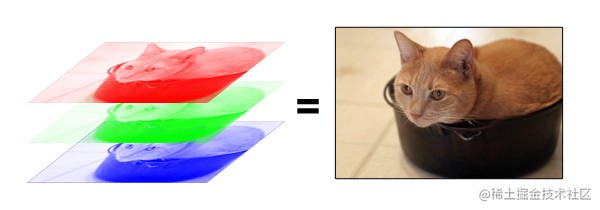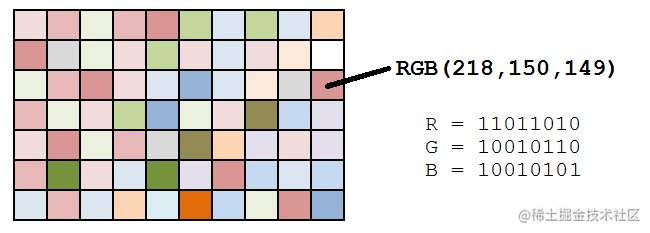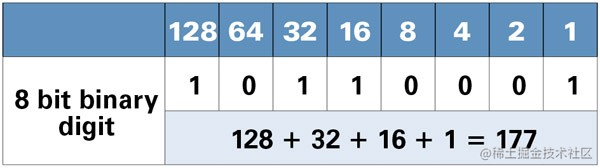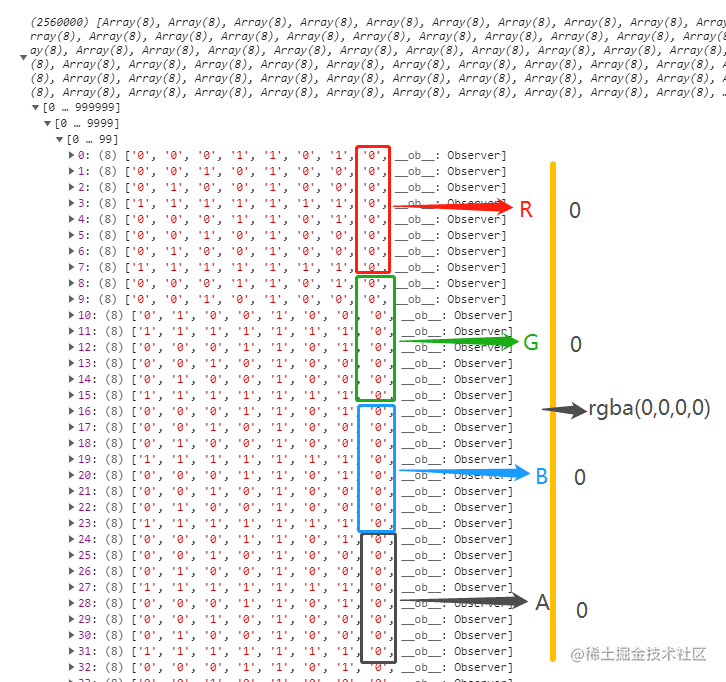### 拿到需要隐藏的数据

(这里为什么要这么做?还是因为上面提到的 主画布的 640000 个像素值的最低位只能储存 80000 个像素的 RGBA 值)

``````//将画布上的信息绘制到小画布上保存起来
saveHiddenImageData() {
const tempCanvas = document.createElement("canvas");
const tempCtx = tempCanvas.getContext("2d");
//小画布的长宽=大画布的像素/8后再开平方
//因为需要八个像素的最低位才可以表示一个小画布的像素的RGBA值
tempCanvas.width = Math.floor(Math.sqrt((this.canvas.width * this.canvas.height) / 8));
tempCanvas.height = Math.floor(Math.sqrt((this.canvas.width * this.canvas.height) / 8));
var image = new Image();
image.src = this.canvas.toDataURL("image/png");
//绘制图像到临时的小画布
tempCtx.drawImage(image, 0, 0, tempCanvas.width, tempCanvas.height);
this.hiddenData = tempCtx.getImageData(0, 0, tempCanvas.width, tempCanvas.height);
this.hiddenData.binaryList = Array.from(this.hiddenData.data, (color) => {
return color;
});
console.log(this.hiddenData, "hiddenData");
this.\$message({
type: "success",
message: "保存成功!请选择目标图片~",
});
this.canvas.clear();
};
},

### 再拿目标图的数据

``````//获取画布像素数据
getCanvasData() {
this.targetData = this.ctx.getImageData(0, 0, this.canvas.width, this.canvas.height);
//将数字化为非奇数
function evenNum(num) {
num = num > 254 ? num - 1 : num;
num = num % 2 == 1 ? num - 1 : num;
return num;
}
//存一个二进制的数值表示
this.targetData.binaryList = Array.from(this.targetData.data, (color, index) => {
this.targetData.data[index] = evenNum(this.targetData.data[index]);
return color;
});
console.log(this.targetData);
},

### 写入隐藏的数据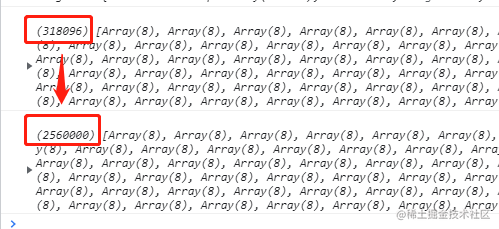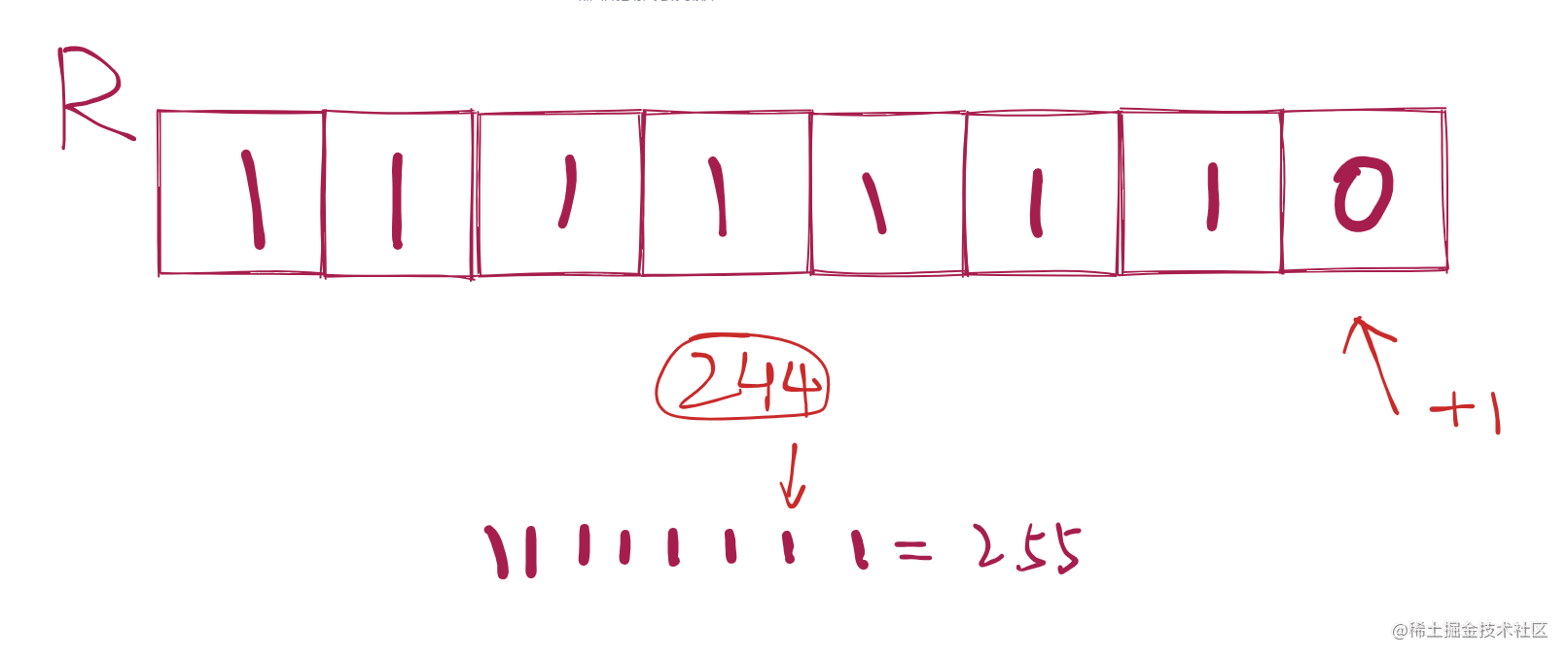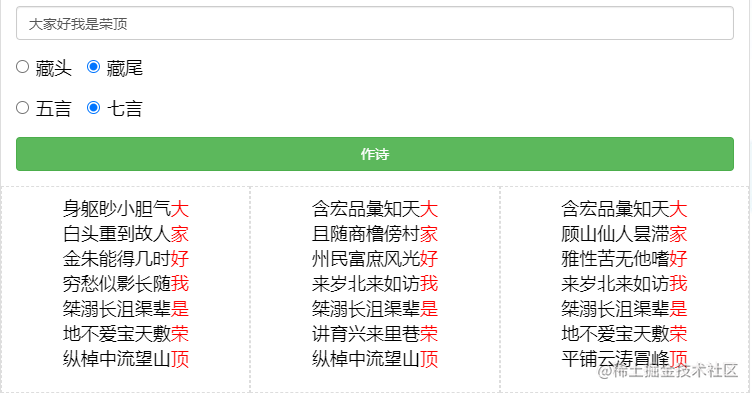RGB 分量值的小量变动，是肉眼无法分辨的，不影响对图片的识别。你看不出来这个+1 的区别

``````//将隐写的资源图片数据存到目标图片的二进制最低位中
drawHiddenData() {
//将隐藏的数据的二进制全部放到一个数组里面
let bigHiddenList = [];
for (let i = 0; i < this.hiddenData.binaryList.length; i++) {
bigHiddenList.push(...this.hiddenData.binaryList[i]);
}
console.log(bigHiddenList, "bigHiddenList");
this.targetData.binaryList.forEach((item, index) => {
bigHiddenList[index] && (item = bigHiddenList[index]);
});
this.canvas.clear();
this.targetData.data.forEach((item, index) => {
this.targetData.data[index] = parseInt(
this.targetData.binaryList[index].join(""),
2
);
});

const tempCanvas = document.createElement("canvas");
tempCanvas.width = 800;
tempCanvas.height = 800;
let ctx = tempCanvas.getContext("2d");
ctx.putImageData(this.targetData, 0, 0);
fabric.Image.fromURL(tempCanvas.toDataURL(), (i) => {
this.canvas.clear();
this.canvas.renderAll();
});
this.\$message({
type: "success",
message: "加密成功!",
});
},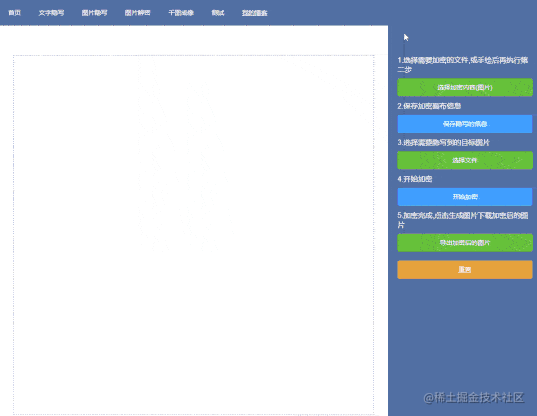### 解析加密后的图片

``````//获取画布像素数据
getCanvasData() {
this.targetData = this.ctx.getImageData(0, 0, this.canvas.width, this.canvas.height);
//存一个二进制的数值表示
this.targetData.binaryList = Array.from(this.targetData.data, (color, index) => {
return color;
});
console.log(this.targetData);
},

### 冲图片中抽取隐藏的颜色值

``````//解析图片
decryptImage() {
const c = document.getElementById("decryptCanvas");
const ctx = c.getContext("2d");

let decryptImageData = [];

for (let i = 0; i < this.targetData.binaryList.length; i += 8) {
let tempColorData = [];
for (let j = 0; j < 8; j++) {
tempColorData.push(this.targetData.binaryList[i + j]);
}
decryptImageData.length < Math.pow(Math.floor(Math.sqrt(2560000 / 32)), 2) * 4 &&
decryptImageData.push([...tempColorData]);
}
decryptImageData = Uint8ClampedArray.from(decryptImageData, (z) => {
z = parseInt(z.join(""), 2);
return z;
});
console.log(decryptImageData, "decryptImageData");
//需要注意的是putImageData的data的长度必须为两个边的乘积的4的倍数
ctx.putImageData(
new ImageData(
decryptImageData,
Math.floor(Math.sqrt(2560000 / 8 / 4)),
Math.floor(Math.sqrt(2560000 / 8 / 4))
),
0,
0
);
},**不要用隐写做违法犯罪的坏事!!!**因为它是可以防（某墙）监控的,比如你把坏孩子图藏进了好孩子图里边,我去太坏了 😢